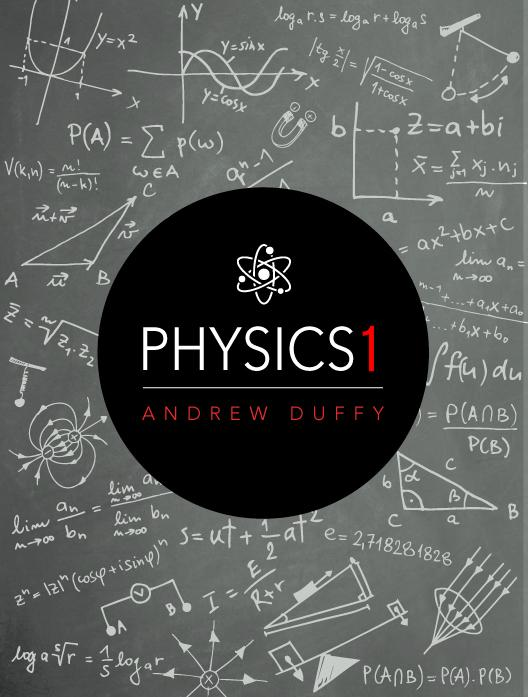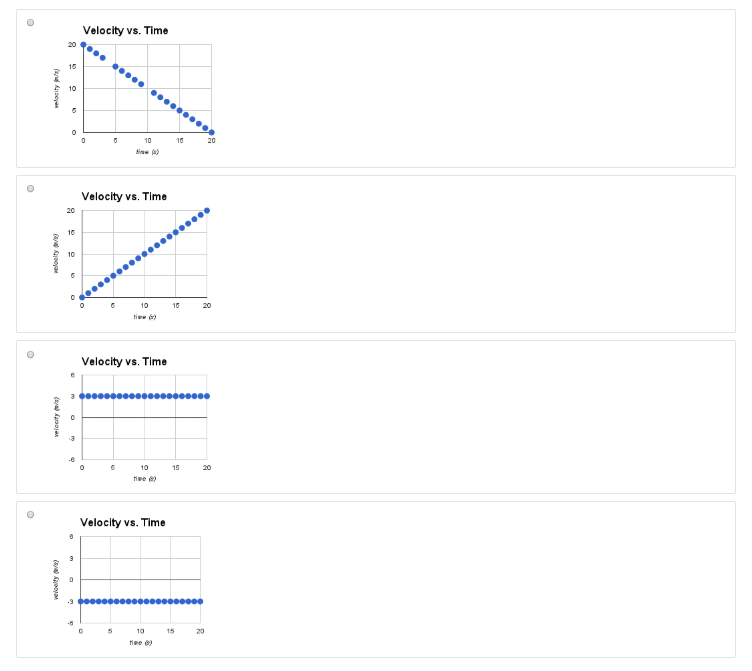# Physics 1

Physics 1 is an interactive e-book covering topics in a typical first-semester physics course, ranging from mechanics to thermodynamics.

# Constant/Average Speed

## Identifying Constant Speed

### Constant speed

Question 1

Let's begin by comparing three different motions. The simulation above shows three different objects moving towards the right. Every two seconds, the simulation leaves a dot at the object's location.

Which object moves at constant speed?

A

Red

B

Blue

C

Black

## Calculating Speed

When an object moves in 1-dimension at constant speed, it moves the same amount of distance for each time interval.

For example, if an object is moving at 5 meters/second, it moves 5 meters every second.

Calculate Speed

Speed is the magnitude of the rate of change in position.  An object's average speed can be calculated by dividing the distance traveled by the time interval in which the travel occurred:

$\overline v=\frac{\mathrm{distance}}{\mathrm{time}}$

When moving at constant speed, the average speed is equal to the constant speed

Example Problem

At the 2013 World Championships in Moscow, Usain Bolt ran 100.0 meters in 9.77 seconds.  What was his average speed?

Given Information:  distance = 100.0 m; time = 9.77 seconds;

Unknown: average speed

$Equation:\;\;\overline v=\frac{\mathrm{distance}}{\mathrm{time}}$

$Solution:\;\overline v=\frac{100.0\;m}{9.77\;s}\Rightarrow\overline v=10.24\;\frac ms$

### Matching Motion

Question 2

Let's now practice identifying motion with another simulation. In this simulation, the dots are left behind at intervals of exactly 1.0 seconds.

Review the situation above and, for each object, use the pull-down menu to select the type of motion it is experiencing. Red:

A

Constant Speed

B

Slowing Down

C

Getting Faster

Question 3

Review the situation above and, for each object, use the pull-down menu to select the type of motion it is experiencing. Blue:

A

Constant Speed

B

Slowing Down

C

Getting Faster

Question 4

Review the situation above and, for each object, use the pull-down menu to select the type of motion it is experiencing. Black:

A

Constant Speed

B

Slowing Down

C

Getting Faster

### Calculation Problem

Question 5

Use the simulation above to respond to the question below.

What is the speed of the object moving at constant speed? _______ m/s

## Speed vs. Velocity (What's the Difference?)

Until now, we have focused our discussion on speed.  However, in physics, we often are more concerned with velocity.  Begin this lesson by playing the animation below.

As you could see, the red object moves to the right while the blue object moves to the left.  Because they meet at the midpoint between their starting points, both objects are moving at the same speed.  However, each object has a different velocity.

Velocity combines speed and direction.  It includes how fast the object is going plus which direction it is moving.

For 1-dimensional motion, we can calculate average velocity by setting up a coordinate system (e.g., to the right is positive) and modifying the average speed equation:

$\overline v=\frac{\mathrm{net}\;\mathrm{displacement}}{\mathrm{time}}=\frac{\triangle x}{\triangle t}=\frac{x_2-x_1}{t_2-t_1}$

If you set to the right as positive, objects moving to the right will have positive velocity while objects moving to the left will have negative velocity.

SCALAR and VECTOR

Speed and velocity are examples of two different types of quantities - scalar and vector.

• Quantities with just a magnitude (and no direction), such as speed, are scalar quantities.
• Quantities with magnitude and direction, such as velocity, are vector quantities.

### Calculate Velocity

Question 6

Let's apply this new idea to the animation above.

What is the velocity of the blue object?

A

-20 m/s

B

-10 m/s

C

-5 m/s

D

0 m/s

E

5 m/s

F

10 m/s

G

20 m/s

Question 7

What is the velocity of the red object?

A

-20 m/s

B

-10 m/s

C

-5 m/s

D

0 m/s

E

5 m/s

F

10 m/s

G

20 m/s

## Graphing Constant Velocity

When an object is moving at constant velocity, the position vs. time graph exhibits a linear relationship (the velocity is the slope of the graph). When the object starts at a point other than the origin, the position vs. time graph is still linear.  The only difference is that, at time = 0 s, the object is at a starting point other than the origin.  This shifts the line up or down depending on the starting location (x0).

In this case, the equation is slighly more complicated.  It still follows the general equation but the x0 does not equal zero.  For this data, the equation will be:

x(t) = 3t + 20

### Velocity vs. Time Graphs?

Question 8

We have looked at position vs. time graphs. Before moving on to non-constant velocity, let's consider what the velocity vs. time graph will look like for the simulation you just looked at.

Which of the following graphs would be the velocity vs. time graph corresponding to the position vs. time graph above?A

a

B

b

C

c

D

d

Explanation

Because the object is moving at constant velocity, the graph should be a straight horizontal line, indicating the same velocity the whole time. Because the velocity is positive, the correct choice is the third one.

## Average Speed Problems

By now you have probably become familiar with using the average speed equation to calculate average speed.  For instance, professional sprinters can run 100 meters in 10 seconds.

$v=\frac{\triangle x}{\triangle t}=\frac{100\;m}{10\;s}=10\frac ms$

You can also use the equation to determine change in position (∆x) or change in t (∆t):

$\begin{array}{l}\boldsymbol S\boldsymbol o\boldsymbol l\boldsymbol v\boldsymbol i\boldsymbol n\boldsymbol g\;\boldsymbol f\boldsymbol o\boldsymbol r\;\boldsymbol\triangle\boldsymbol x:\;\;v=\frac{\triangle x}{\triangle t}\rightarrow\triangle t\left(v\right)=\left(\frac{\triangle x}{\triangle t}\right)\triangle t\rightarrow\boldsymbol\triangle\boldsymbol x\boldsymbol=\boldsymbol v\boldsymbol\triangle\boldsymbol t\\\boldsymbol S\boldsymbol o\boldsymbol l\boldsymbol v\boldsymbol i\boldsymbol n\boldsymbol g\;\boldsymbol f\boldsymbol o\boldsymbol r\;\boldsymbol\triangle\boldsymbol t\boldsymbol:\;\;v=\frac{\triangle x}{\triangle t}\rightarrow\frac1v\left(\triangle x\right)=\left(v\triangle t\right)\frac1v\rightarrow\boldsymbol\triangle\boldsymbol t\boldsymbol=\frac{\boldsymbol\triangle\mathbf x}{\mathbf v}\\\end{array}$

### Calculating Speed

Question 9

The top runners in the Boston Marathon complete the 26.2 miles in about 2 hours and 10 minutes.

If 1609 meters = 1 mile, what is the marathoner's speed in meters/second? _______ m/s

### Calculating Position

Question 10

Professional sprinters can sprint at 10 m/s.

How far (in miles) would a professional sprinter travel if they could maintain the same speed for 2 hours and 10 minutes? _______ miles

### Calculating Time

Question 11

Humans can walk at an average speed of 2.00 m/s.

How many hours would it take someone to walk the 4800 km from New York to Los Angeles? _______ hours

### Challenge Question

Question 12

Two trains are 150 km apart. Train A moves at 50.0 km/hr towards train B, which moves at 30.0 km/hr toward train A on the same track.

How much time do they have before the trains collide? _______ hours

Transcripts

## Figure References

All images created by author using Keynote.

All simulations constructed using HTML5/Javascript.

All videos created by author using Keynote.